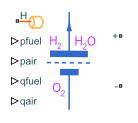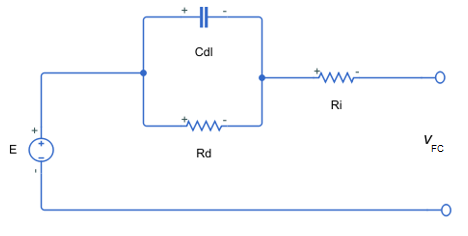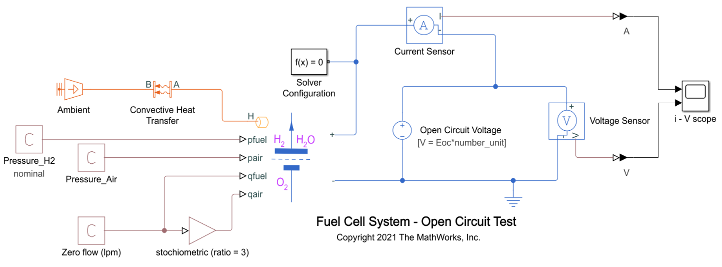# Fuel Cell

Fuel cell electrical system

Since R2021a

•Libraries:
Simscape / Electrical / Sources

## Description

The Fuel Cell block models a fuel cell that converts the chemical energy of hydrogen into electrical energy.

This chemical reaction defines the electrical conversion:

`${H}_{2}+\frac{1}{2}{O}_{2}\to {H}_{2}O+heat$`

which occurs due to these anode and cathode half-reactions:

`$\begin{array}{l}{H}_{2}\to 2{H}^{+}+2{e}^{-}\\ \frac{1}{2}{O}_{2}+2{H}^{+}+2{e}^{-}\to {H}_{2}O\end{array}$`A fuel cell stack comprises several series-connected fuel cells. This figure shows the equivalent circuit of a single fuel cell that this block useswhere:

• VFC is the cell voltage.

• Ri is the Internal resistance.

• Rd is the Sum of activation and concentration resistances.

• Cdl is the parallel RC capacitance that accounts for time dynamics in the cell.

### Equations

You can use the Model fidelity parameter to set the Fuel Cell block to two different levels of fidelity:

• `Simplified - nominal conditions` — The block calculates the Nernst voltage at the nominal condition of temperature and pressure.

• `Detailed with physical inputs` — The block calculates the Nernst voltage by considering the pressures and flow rates of fuel and air.

Simplified Electrical Model

When Model fidelity is set to ```Simplified - nominal conditions```, the Fuel Cell block calculates the Nernst voltage, E, at the nominal condition of temperature and pressure, as defined by these equations:

`$\begin{array}{l}E={E}_{oc}-NAln\left(\frac{{i}_{FC}}{{i}_{0}}\right)\\ {v}_{FC}={N}_{unit}E-{R}_{i}{i}_{FC}-{v}_{d}\\ \frac{1}{{R}_{d}}\left(\tau \frac{d{v}_{d}}{dt}+{v}_{d}\right)={i}_{FC}\end{array}$`

where:

• Eoc is the Open-circuit voltage.

• N is the Number of cells per module.

• iFC is the current that the fuel cell generates.

• vFC is the voltage across the fuel cell terminals.

• Nunit is the Module units (Series).

• vd is the voltage drop that accounts for fuel cell dynamics.

• A is the Tafel slope, in volts.

• i0 is the Nominal exchange current.

• $\tau ={R}_{d}{C}_{dl}$.

Detailed Electrical Model

When Model fidelity is set to ```Detailed with physical inputs```, the Fuel Cell block calculates the Nernst voltage, E, by considering the pressures and flow rates of fuel and air.

These equations determine the rates of utilization of hydrogen, UH2, and oxygen, UO2

`$\begin{array}{l}{U}_{{H}_{2}}=\frac{60000N{i}_{FC}{V}_{e}}{{p}_{fuel}{q}_{fuel}{x}_{{H}_{2}}}\\ {U}_{{O}_{2}}=\frac{60000N{i}_{FC}{V}_{e}}{2{p}_{air}{q}_{air}{x}_{{O}_{2}}}\end{array}$`

where:

• Ve is the thermal voltage at room temperature.

• pfuel is the supply pressure of the fuel, in `bar`.

• qfuel is the fuel flow rate.

• xH2 is the concentration of hydrogen in the fuel, in percent.

• pair is the supply pressure of air, in `bar`.

• qair is the air flow rate.

• xO2 is the concentration of oxygen in the air, in percent.

These equations define the partial pressures:

`$\begin{array}{l}{p}_{{H}_{2}}={p}_{fuel}{x}_{{H}_{2}}-{U}_{{H}_{2}}\\ {p}_{{O}_{2}}={p}_{air}{x}_{{O}_{2}}-{U}_{{O}_{2}}\\ {p}_{{H}_{2}O}={p}_{air}{x}_{{H}_{2}O}-2{U}_{{O}_{2}}\end{array}$`

where xH2O is the concentration of vapor in air, in percent.

Then, the block computes the Nernst voltage aswhere:

• ${K}_{z}=\frac{\frac{{E}_{oc_adm}}{{K}_{c}N}-1.229}{\left({T}_{nom}-298\right)\frac{-44.43}{{z}_{0}F}+\frac{R{T}_{nom}}{{z}_{0}F}\mathrm{ln}\left({p}_{nH2}{p}_{n{O}_{2}}^{\frac{1}{2}}\right)}$.

• ${E}_{Tafel}=N{A}_{T}ln\left(\frac{{i}_{FC}}{{i}_{0}}\right)$ is the electrokinetic term for the activation.

• ${E}_{conc}={V}_{e}\frac{T}{298}\mathrm{ln}\left(\frac{{i}_{lim}}{{i}_{lim}-{i}_{FC}}\right)$ is the electrokinetic term for the concentration.

• ${E}_{oc_adm}=max\left({E}_{oc},N\left(1.229+\frac{R{T}_{nom}}{2F}\mathrm{ln}\left({p}_{n{H}_{2}}{p}_{n{O}_{2}}^{\frac{1}{2}}\right)\right)\right)$.

• Kc is the voltage constant at nominal condition of operation.

• T is the operating temperature of the fuel cell.

• Tnom is the value of the Nominal temperature parameter.

• z is the number of moving electrons per second.

• z0 is the number of moving electrons per second at the value of the Nominal exchange current parameter.

• F is the Faraday constant.

• R is the universal gas constant.

• pnH2 is the nominal hydrogen pressure, in `bar`.

• pnO2 is the nominal oxygen pressure, in `bar`.

• ${A}_{T}=\frac{AT}{\mathrm{ln}\left(10\right)\ast 298}$ is the Tafel slope as a function of the temperature.

• ilim is the value of the Collapse current parameter.

• The voltage `1.229` represents the standard cell potential for the Nernst equation.

The block computes the power dissipated, or the heat released in the fuel cell, by using this equation

`${P}_{dissipated}={Z}_{e_agg}\left(T\Delta S\right)+{R}_{i}{i}_{FC}+{v}_{d}^{2}\frac{1}{{R}_{d}}$`

where:

• ${Z}_{e_agg}=\frac{\left({N}_{unit}E-{R}_{i}{i}_{FC}-{v}_{d}\right){i}_{FC}}{2\Delta G}$ is the total electron circulation rate, in `mol/s`.

• TΔS is the change in entropy of the fuel cell reaction, in `kJ/(mol*K)`, at the operating temperature of the fuel cell.

• ΔG is the change in Gibbs free energy of the full fuel cell reaction, in `kJ/mol`, at the operating temperature of the fuel cell.

### Variables

To set the priority and initial target values for the block variables prior to simulation, use the Initial Targets section in the block dialog box or Property Inspector. For more information, see Set Priority and Initial Target for Block Variables.

Nominal values provide a way to specify the expected magnitude of a variable in a model. Using system scaling based on nominal values increases the simulation robustness. Nominal values can come from different sources, one of which is the Nominal Values section in the block dialog box or Property Inspector. For more information, see System Scaling by Nominal Values.

## Limitations

The Fuel Cell block does not allow electrolysis.

## Ports

### Input

expand all

Physical signal port associated with the supply absolute pressure of the fuel, in `bar`, specified as a physical signal.

#### Dependencies

To enable this port, set Model fidelity to `Detailed with physical inputs`.

Physical signal port associated with the supply gauge pressure of air, in `bar`, specified as a physical signal.

#### Dependencies

To enable this port, set Model fidelity to `Detailed with physical inputs`.

Physical signal port associated with the fuel flow rate, specified as a physical signal.

#### Dependencies

To enable this port, set Model fidelity to `Detailed with physical inputs`.

Physical signal port associated with the air flow rate, specified as a physical signal.

#### Dependencies

To enable this port, set Model fidelity to `Detailed with physical inputs`.

### Conserving

expand all

Electrical conserving port associated with the fuel cell positive terminal.

Electrical conserving port associated with the fuel cell negative terminal.

Thermal conserving port.

#### Dependencies

To enable this port, set Model fidelity to `Detailed with physical inputs`.

## Parameters

expand all

### Main

Level of fidelity of the fuel cell model.

Open-circuit voltage.

When the amount of flow is very low or close to zero and the values of the fuel and air pressures are equal to their nominal values, the output voltage of the fuel cell is equal to the open-circuit voltage multiplied by the number of module units. The current that flows out of the fuel cell is negligible. This figure shows how to set the open-circuit test:Amount of overpotential required to increase the reaction rate by a factor ten.

Internal resistance.

Exchange current at the nominal temperature.

At the nominal exchange current, the fuel cell leaves the region of activation polarization and enters the region of ohmic polarization.

Value of current at which the fuel cell voltage becomes zero. As the fuel cell enters the region of concentration polarization and the current continues to increase, the voltage starts decreasing at a faster rate.

#### Dependencies

To enable this parameter, set Model fidelity to `Detailed with physical inputs`.

Number of cells per module.

The cell count value in this block is consistent with the fuel cell delivering its maximum output power for specified set points of flow and H2 pressure.

Stack of module units in series.

Stack module in series to scale up voltage. For example, 10 module units in series with an open-circuit voltage of 65 V deliver a voltage of 650V.

### Supply

To enable these parameters, in the Main tab, set Model fidelity to ```Detailed with physical inputs```.

Hydrogen gauge pressure at nominal temperature.

#### Dependencies

To enable this parameter, set Model fidelity to `Detailed with physical inputs`.

Oxygen gauge pressure at nominal temperature.

#### Dependencies

To enable this parameter, set Model fidelity to `Detailed with physical inputs`.

Molar fraction concentration of hydrogen in fuel, in percent.

#### Dependencies

To enable this parameter, set Model fidelity to `Detailed with physical inputs`.

Molar fraction concentration of oxygen in fuel, in percent.

#### Dependencies

To enable this parameter, set Model fidelity to `Detailed with physical inputs`.

Molar fraction concentration of vapor in air, in percent.

#### Dependencies

To enable this parameter, set Model fidelity to `Detailed with physical inputs`.

### Dynamics

Whether to model the activation delay of the fuel cell.

Sum of the activation resistance and concentration resistance.

#### Dependencies

To enable this parameter, set Model activation delay to `Yes`.

Time constant.

#### Dependencies

To enable this parameter, set Model activation delay to `Yes`.

### Thermal

To enable these parameters, in the Main tab, set Model fidelity to ```Detailed with physical inputs```.

Temperature at which the nominal parameters are measured.

#### Dependencies

To enable this parameter, set Model fidelity to `Detailed with physical inputs`.

Thermal mass associated with the thermal port, H. This value represents the energy required to raise the temperature of the thermal port by one degree.

#### Dependencies

To enable this parameter, set Model fidelity to `Detailed with physical inputs`.

 Do, T.C., et al. “Energy Management Strategy of a PEM Fuel Cell Excavator with a Supercapacitor/Battery Hybrid Power Source”. Energies 12, no. 22, (November 2019). DOI.org (Crossref), doi:10.3390/en13010136.

 Motapon, Souleman N., O. Tremblay and L. Dessaint, “A generic fuel cell model for the simulation of fuel cell vehicles.” 2009 IEEE Vehicle Power and Propulsion Conference, Dearborn, MI, 2009, pp. 1722-1729, doi: 10.1109/VPPC.2009.5289692

 Hirschenhofer, J. H.,, D.B. Stauffer, R.R. Engleman, and M.G. Klett. Fuel Cell Handbook (4th Ed). U.S. Department of Energy Office of Fossil Energy, 1988.

 Larminie, James, and Andrew Dicks. Fuel Cell Systems Explained. West Sussex, England: John Wiley & Sons, Ltd,., 2003. https://doi.org/10.1002/9781118878330.